# KSEEB SSLC Class 10 Science Solutions Chapter 12 Electricity

KSEEB SSLC Class 10 Science Solutions Chapter 12 Electricity are part of KSEEB SSLC Class 10 Science Solutions. Here we have given Karnataka SSLC Class 10 Science Solutions Chapter 12 Electricity.

## Karnataka SSLC Class 10 Science Solutions Chapter 12 Electricity

### KSEEB SSLC Class 10 Science Chapter 12 Intext Questions

Text Book Part I Page No. 94

Question 1.
What does an electric circuit mean?
A continuous and closed path of an electric current is called an electric circuit.

Question 2.
Define the unit of current.
The SI unit of electric current is ampere (A).
When I coulomb of electric charge flows through any cross. Section of a conductor in I second, the electric current flowing through it is said to be 1 ampere.
∵ 1 ampere = 1C/1s

Question 3.
Calculate the number of electrons constituting one coulomb of charge.
The SI unit of electric charge is column, which is equivalent to the charge contained in nearly 6 × 1018 electrons.

Text Book Part I Page No. 96

Question 1.
Name a device that helps to maintain a potential difference across a conductor.
Voltmeter.

Question 2.
What is meant by saying that the potential difference between two points is 1 V?
One Volt is the potential difference between two points in a current-carrying conductor when 1 joule of work is done to move a charge of 1 coulomb from one point to the other.1 V = 1 Jc-1

Question 3.
How much energy is given to each coulomb of charge passing through a 6 V battery?
W = VQ
= 6 × 1 = 6 joules
Hence 6 joules of energy is given to each coulomb of charge passing through a 6 V battery.

Text Book Part I Page No. 103

Question 1.
On what factors does the resistance of a conductor depend?
The resistance of the conductor depends

1. on its length
2. on its area of cross section and
3. on the nature of its material.

Question 2.
Will current flow more easily through a thick wire or a thin wire of the same material, when connected to the same source? Why?
Resistance, $$\mathrm{R} \alpha \frac{1}{\mathrm{A}}$$. The resistance of a conductor is inversely proportional to its area of cross-section. A thick wire has a greater area of cross-section whereas a thin wire has a smaller area of cross-section. Thus, thick wire has less resistance and a thin wire has more resistance therefore current will flow more easily through a thick wire.

Question 3.
Let the resistance of an electrical component remains constant while the potential difference across the two ends of the component decreases to half of its former value. What change will occur in the current through it?
As per ohm’s law, V = IR
$$\mathrm{I}=\frac{\mathrm{V}}{\mathrm{R}}$$ —— (i)
Potential difference is half∴ Current flowing is also half of ts former value.

Question 4.
Why are coils of electric toasters and electric irons made of an alloy rather than a pure metal?
a) Resistivity of iron = 10.0 × 10-8
Resistivity of Mercury = 94.0 × 10-8
Resistivity of an alloy is greater than iron. By this we conclude that, Iron is good conductor of heat comparing to Mercury.
b) Resistivity of silver is less, hence it is a good conductor of heat.

Text Book Part I Page No. 107

Question 1.
Draw a schematic diagram of a circuit consisting of a battery of three cells of 2 V each, a 5 Ω resistor, an 8Ω resistor, and a 12Ω resistor, and a plug key, all connected in series.
Rs = R1 + R2 + R3
= 2V + 2V + 2V
= 6WQuestion 2.
Redraw the circuit of Question 1, putting in an ammeter to measure the current through the resistors and a voltmeter to measure the potential difference across the 12Ω resistor. What would be the readings in the ammeter and the voltmeter?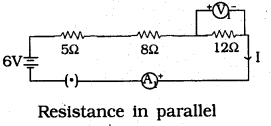Resistance, R = 5 + 8 + 12 = 25 Ω
$$\mathrm{I}=\frac{\mathrm{V}}{\mathrm{R}}=\frac{6}{25}=0.24 \mathrm{A}$$
As per ohm’s law =0.24A
V1 = IR
Potential difference
V1 = IR = 0.24 × 12 = 2.88 V.
reading in voltmeter = 2.88 v.

Text Book Part I Page No. 110

Question 1.
Judge the equivalent resistance when the following are connected in parallel –
(a) 1 Ω and 106 Ω,
(b) 1 Ω and 103 Ω, and 106 Ω.
(a) If two resistances are connected in parallelequivalent resistance = 1Ω.
(b) If three resistances 1Ω, 103Ω and 106Ω are connected in parallel,equivalent resistance = 0.999Ω

Question 2.
An electric lamp of 100Ω, a toaster of resistance 50Ω and a water filter of resistance 500Ω are connected in parallel to a 220 V source. What is the resistance of an electric iron connected to the same source that takes as much current as all three appliances, and what is the current through it?
Resistance of Electric 1 amp R1 = 100Ω
Resistance of Tosser, R2 = 50Ω
Resistance of water filter, R3 = 50Ω
Potential difference, V = 220V
When these are connected in parallel,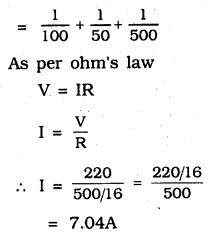7.04A of electricity is obtained by three appliances
Resistance of an electric iron connected to the same source that takes as much current as all three appliances∴ Resistance of iron box = 31.25Ω
Electricity flowing through this = 7.04A.

Question 3.
What are the advantages of connecting electrical devices in parallel with the battery instead of connecting them in series?
(i) In parallel circuit, if one electrical appliance stops working due to some defect, then all other appliances keep working normally. In series circuit, if one electrical appliance stops working due to some defect, then all other appliances also stop working.

(ii) In parallel circuits, each electrical appliance gets the same voltage as that of the power supply line. In series circuit, appliances do not get the same voltage, as that of the power supply line.

(iii) In the parallel connection of electrical appliances, the overall resistance of the household circuit is reduced due to which the current from the power supply is high. In the series connection, the overall resistance of the circuit increases too much due to which the current from the power supply is low.

Question 4.
How can three resistors of resistances 2Ω, 3Ω, and 6Ω be connected to give a total resistance of (a) 4Ω, (b) 1Ω?
In this diagram 2 resistors of resistance 3W and 3 W are connected in parallel.If 2Ω and 2Ω are connected in series 2Ω + 2Ω = 4Ω
∴ Total resistance = 4Ω
If resistors are connected in series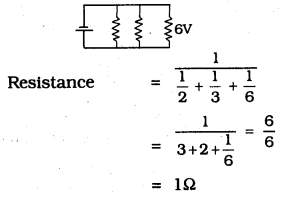∴ Total resistance = 1Ω

Question 5.
What is (a) the highest, (b) the lowest total resistance that can be secured by combinations of four coils of resistance 4Ω, 8Ω, 12Ω, 24Ω?
(a) If four resistors are connected in series then resistance
= 4 + 8 + 12 + 24
= 48Ω
(b) If these are connected in parallel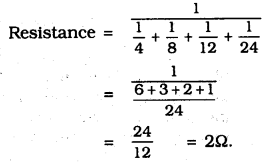∴ We can get lowest resistance.

Text Book Part I Page No. 112

Question 1.
Why does the cord of an electric heater not glow while the heating element does?
In cord of an electric heater, as current flows these become hot arid glows but in case of electric heater this will not happen.

Question 2.
Compute the heat generated while transferring 96000 coulomb of charge in one hour through a potential difference of 50 V.
As per Joule’s law H = VIt.
Here V = 50 V.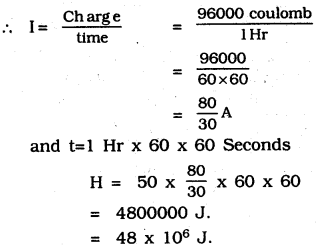Question 3.
An electric iron of resistance 20Ω takes a current of 5 A. Calculate the heat developed in 30 s.
As per Joule’s law
H = VIt
H = IR = 5A × 20Ω = 100V
I = 5A, t = 30 sec.
∴ H = 100 × 5 × 30 J
= 15000 J = 1.5 × 104 J.

Text Book Part I Page No. 114

Question 1.
What determines the rate at which energy is delivered by a current?
The rate at which electric energy is dissipated or consumed in an electric circuit is termed as electric power.
P = VI.

Question 2.
An electric motor takes 5 A from a 220 V line. Determine the power of the motor and the energy consumed in 2 h.
P = VI
V = 220V, and I = 5A.
Power P = 220 × 5 = 1100 W
Power of the motor = P × t
P = 1100 W.
t = 2 Hr 2 × 60 × 60 W
= 7200 S
∴ Energy consumed, E = 1100 × 7200 J
= 7920000
= 7.92 × 106J.

### KSEEB SSLC Class 10 Science Chapter 12 Textbook Exercises

Question 1.
A piece of wire of resistance R is cut into five equal parts. These parts are then connected in parallel. If the equivalent resistance of this combination is R’, then the ratio $$\frac { R }{ R` }$$ is –
(a) $$\frac { 1 }{ 25 }$$
(b) $$\frac { 1 }{ 5 }$$
(c) 5
(d) 25
(d) 25

Question 2.
Which of the following terms does not represent electrical power in a circuit?
(a) I2R
(b) IR2
(c) VI
(d) $$\frac { { V }^{ 2 } }{ R }$$
(b) IR2

Question 3.
An electric bulb is rated 220 V and 100 W. When it is operated on 110 V, the power consumed will be –
(a) 100 W
(b) 75 W
(c) 50 W
(d) 25 W
(d) 25 W

Question 4.
Two conducting wires of the same material and of equal lengths and equal diameters are first connected in series and then parallel in a circuit across the same potential difference. The ratio of heat produced in series and parallel combinations would be-
(a) 1 : 2
(b) 2 : 1
(c) 1 : 4
(d) 4 : 1
(c) 1 : 4

Question 5.
How is a voltmeter connected in the circuit to measure the potential difference between two points?
Voltmeter is connected in parallel in the circuit to measure the potential difference between two points.

Question 6.
A copper wire has diameter 0.5 mm and resistivity 1.6 10-8Ω m. What will be the length of this wire to make its resistance 10Ω? How much does the resistance change if the diameter is doubled?
Area of the copper wire A $$=\pi\left(\frac{\mathrm{D}}{2}\right)^{2}$$
diameter = 0.5 mm = 0.0005 m
Resistance, R = 10W.Diameter of copper wire is doubled, then diameter = 2 × 0.5 = 1 mm = 0.001 m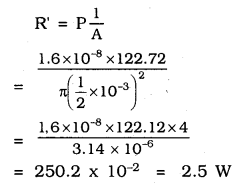∴ Length of wire = 122.7 m
Resistance of wire = 2.5 W

Question 7.
The values of current I flowing in a given resistor for the corresponding values of potential difference V across the resistor are given below –

 I (amperes) 0.5 1 2 3 4 V (volts) 1.6 3.4 6.7 10.2 13.2

Plot a graph between V and I and calculate the resistance of that resistor.
In the following graph, voltage is taken along the x-axis and the current is taken along the y-axis. Different values are as follows:

 V (volts) 1.6 3.4 6.7 10.2 13.2 I (amperes) 0.5 1 2 3 4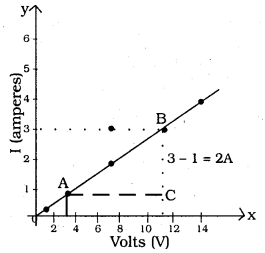Slope indicates Resistance∴ Resistance of the resistor = 3.4Ω.

Question 8.
When a 12 V battery is connected across an unknown resistor, there is a current of 2.5 mA in the circuit. Find the value of the resistance of the resistor.
Here I = 2.5 mA = 2.5 × 10-3 A.
∴ $$R=\frac{12}{2.5 \times 10^{-3}}$$
= 4.8 × 103
= 4.8 K cal.

Question 9.
A battery of 9 V is connected in series with resistors of 0.2Ω, 0.3Ω, 0. 4Ω, 0.5Ω and 12Ω, respectively. How much current would flow through the 12Ω resistor?
A battery of 9V is connected in series with resistors 0.2Ω, 0.3Ω, 0.4Ω,
R = 0.2 + 0.3 + 0.4 + 0.5 + 12 = 13.4Ω.
V = 9V
$$I=\frac{9}{13.4}=0.671 \mathrm{A}$$
∴ 12 V battery, 0.671 A current flows.

Question 10.
How many 176Ω resistors (in parallel) are required to carry 5 A on a 220 V line?
Let the resistors be x’
Resistance = 176Ω
As per ohm’s law,
V = IR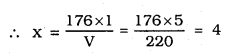∴ Four resistors (in parallel) are required to carry 5A on a 220 V line.

Question 11.
Show how you would connect three resistors, each of resistance 6Ω, so that the combination has a resistance of (i) 9Ω, (ii) 4Ω.
If resistors are connected in series 6Ω + 6Ω + 6Ω =18Ω This ia not correct
When they are connected in parallel
$$\frac{1}{6}+\frac{1}{6}+\frac{1}{6}=3$$ This is also wrong,
i) When they are connected in parallelTwo 6Ω resistors are connected in parallelIf 3rd resistor of 6Ω and 3Ω are connected in series, it becomes 6Ω + 3Ω = 9Ω.
ii) When they are connected in seriesResistance = 6Ω + 6Ω
= 12Ω
If 3rd resistor 6Ω is connected to 12Ω in parallelTotal resistance = 4Ω.

Question 12.
Several electric bulbs designed to be used on a 220 V electric supply line, are rated 10 W. How many lamps can be connected in parallel with each other across the two wires of 220 V line if the maximum allowable current is 5 A?
Here V = 220V
I – 5A∴ 110 lamps can be connected in parallel with each other across the two wires.

Question 13.
A hot plate of an electric oven connected to a 220 V line has two resistance coils A and B, each of 24Ω resistance, which may be used separately, in series, or in parallel. What are the currents in the three cases?
(i) If coils are connected separately V = 220 V
Resistance R1 = 240
As per ohm’s law, V = IR∴ If coils are connected separately 9.16A electricity flows in the coil.
(ii) If coils are connected in series
Resistance R2 = 24Ω + 24Ω = 48Ω
As per ohm’s law V = IR∴ If coils are connected in series 4.58A electricity flows.

Question 14.
Compare the power used in the 20 resistors in each of the following circuits:
(i) a 6 V battery in series with 1Ω and 2Ω resistors, and
(ii) a 4 V battery in parallel with 12Ω and 2Ω resistors.
(i) Potential Difference V = 6V
If 1Ω and 2Ω resistors are connected in series, then Resistance
R = 1 + 2 = 3W.
As per ohm’s law
$$\mathrm{I}=\frac{6}{3}=2 \mathrm{A}$$
P(I2)R = (2)2 × 2 = 8W.
(ii) Potential difference V = 4V
If 12Ω and 2Ω resistors are connected in parallel, voltage is equal
Voltage of resistance 2 W is 4 volts∴ Power of 2Ω is 8 W.

Question 15.
Two lamps, one rated 100 W at 220 V, and the other 60 W at 220 V, are connected in parallel to electric mains supply. What current is drawn from the line if the supply voltage is 220 V?
Both lamps are connected in parallel potential difference = 220 V
Power = V × I.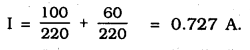Question 16.
Which uses more energy, a 250 W TV set in 1 hr, or a 1200 W toaster in 10 minutes?
250W TV set in used in 1 Hr, then its energy
= 250 × 3600 = 9 × 105
energy of Toaster = 1200 × 600
If it is used in 10 minutes, then its power = 1200 × 600 × 7.2 × 105 J
∴ Energy of 250 W TV set is used in 1 Hr is greater than 1200 W toaster used in 10 minutes.

Question 17.
An electric heater of resistance 8Ω draws 15 A from the service mains 2 hours.
Calculate the rate at which heat is developed in the heater.
P = I2R
R = 8Ω, I = 15A
P= (15)2 × 8 = 1800 J/s.
∴ Rate at which heat is developed in the heater
= 1800 J/s.

Question 18.
Explain the following.
(a) Why is the tungsten used almost exclusively for filament of electric lamps?
Tungstan is a strong and metal having high melting point. This will not melt in high temperature. Because of this tungsten is used almost exclusively for filament of electric lamps.

(b) Why are the conductors of electric heating devices, such as bread- toasters and electric irons, made of an alloy rather than a pure metal?
The resistivity of an alloy is generally higher than that of its constituent metals. Alloys do not oxidise (burn) readily at high temperatures. Because of this reason the conductors of electric heating devices, such as bread-toasters and electric irons made of an alloy rather than a pure metal.

(c) Why is the series arrangement not used-for domestic circuits?
In case of series connection, when one component fails, the circuit it broken and none of the components work. But in case of parallel connection, circuit divides the current throughout the electrical gadgets. Because of this reason series arrangement is not used for domestic purposes.

(d) How does the resistance of a wire vary with its area of cross-section?
Resistance is inversely proportional to its cross section. As area is increasing resistance is less. Thus resistance of wire is changing with area of cross section.

(e) Why are copper and aluminium wires usually employed for electricity transmission?
Copper and Aluminium are good conductors of electricity and they have less resistance. Hence they are used in electricity transmission.

### KSEEB SSLC Class 10 Science Chapter 12 Additional Questions and Answers

Question 1.
Draw a Neat diagram of Electric circuit for studying ohm’s law.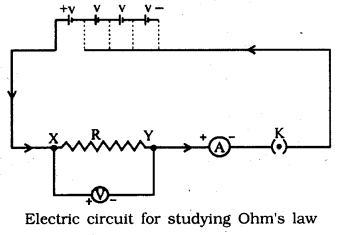Question 2.
What is the S.I. Unit of resistivity?
ΩM

Question 3.
How is fuse wire made of?
It consists of a piece of wire made of a metal or an alloy of appropriate melting point, for example aluminium, copper, iron, lead etc.

Question 4.
Write the formula of Electric power.
$$P\quad =\quad \frac { { V }^{ 2 } }{ R }$$